Next: Worked example 8.5: Hinged Up: Rotational motion Previous: Worked example 8.3: Moment

## Worked example 8.4: Weight and pulley

Question: A weight of mass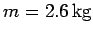is suspended via a light inextensible cable which is wound around a pulley of mass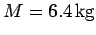and radius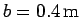. Treating the pulley as a uniform disk, find the downward acceleration of the weight and the tension in the cable. Assume that the cable does not slip with respect to the pulley.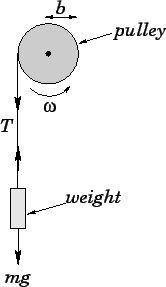Answer: Letbe the instantaneous downward velocity of the weight,the instantaneous angular velocity of the pulley, and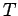the tension in the cable. Applying Newton's second law to the vertical motion of the weight, we obtain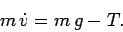The angular equation of motion of the pulley is writtenwhere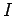is its moment of inertia, and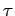is the torque acting on the pulley. Now, the only force acting on the pulley (whose line of action does not pass through the pulley's axis of rotation) is the tension in the cable. The torque associated with this force is the product of the tension,, and the perpendicular distance from the line of action of this force to the rotation axis, which is equal to the radius,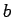, of the pulley. Hence,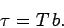If the cable does not slip with respect to the pulley, then its downward velocity,, must match the tangential velocity of the outer surface of the pulley,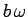. Thus,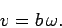It follows that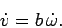The above equations can be combined to give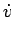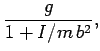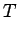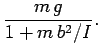Now, the moment of inertia of the pulley is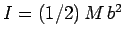. Hence, the above expressions reduce to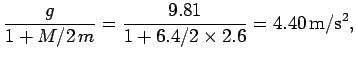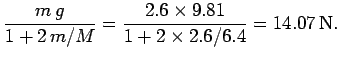Next: Worked example 8.5: Hinged Up: Rotational motion Previous: Worked example 8.3: Moment
Richard Fitzpatrick 2006-02-02